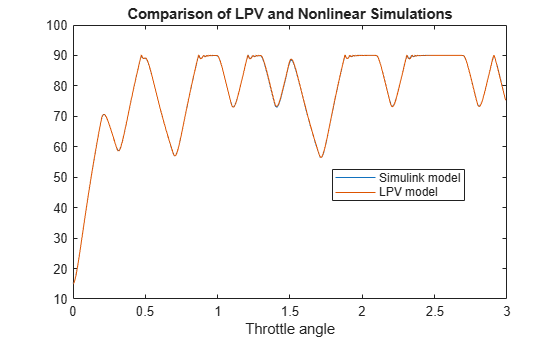# LPV Model of Engine Throttle

This example shows how to model engine throttle behavior as a linear parameter-varying (LPV) model with state offsets ${\stackrel{˙}{\mathit{x}}}_{0}\left(\mathit{p}\right)$ to account for nonlinearity. You also simulate the results of the LPV model and compare the results with nonlinear simulation.

The throttle controls the air mass flow into the intake manifold of an engine. The throttle body contains a butterfly valve that opens when the driver presses down on the accelerator pedal. This lets more air enter the cylinders and causes the engine to produce more torque. The butterfly valve is modeled as a mass-spring-damper system. The amount of rotation of the valve is limited to between 15 degrees and 90 degrees.

### LPV Model

The throttle dynamics are defined in the function `throttleLPV.m` provided with this example.

Use `lpvss` to create the model. This model is parameterized by the throttle angle, which is the first state of the model.

```c0 = 50; k0 = 120; K0 = 1e6; b0 = 4e4; Ts = 0; x0 = [15;0]; DF = @(t,p) throttleLPV(p,c0,k0,b0,K0); sysp = lpvss('p',DF,Ts,0,15);```

### LPV Simulation

Generate open-loop response to an input along a parameter trajectory.

For this model, specify $\mathit{p}\left(\mathit{t}\right)$ implicitly as a function $\mathit{F}\left(\mathit{t},\mathit{x},\mathit{u}\right)$ of time $\mathit{t}$, input $\mathit{u}$, and state $\mathit{x}$. Here $\mathit{p}\left(\mathit{t}\right)$ is the throttle angle (first state).

```load ThrottleInputData.mat t2 = linspace(t(1),t(end),2000); u2 = interp1(t,u,t2); p = @(t,x,u)x(1); yLPV = lsim(sysp,u2,t2,x0,p);```

Plot the response.

```plot(t2,yLPV) title('LPV Simulation') xlabel('Throttle angle')```### Nonlinear Simulation

```open_system('throttleNLModel') ysl = sim('throttleNLModel'); ysl = interp1(ysl.ScopeData(:,1),ysl.ScopeData(:,2),t);```

Compare the simulation results for the LPV and nonlinear models.

```figure(1) clf plot(t,ysl,t2,yLPV) legend('Simulink model','LPV model','location','Best') title('Comparison of LPV and Nonlinear Simulations') xlabel('Throttle angle')```The LPV model simulates the nonlinear response well because the model uses the actual trajectory for scheduling.

Close the model.

`bdclose('throttleNLModel')`

### Data Function

`type throttleLPV.m`
```function [A,B,C,D,E,dx0,x0,u0,y0,Delays] = throttleLPV(x1,c,k,b,K) % LPV representation of engine throttle dynamics. % Ref: https://www.mathworks.com/help/sldo/ug/estimate-model-parameter-values-gui.html % x1: scheduling parameter (throttle angle; first state of the model) % c,k,b,K: physical parameters A = [0 1; -k -c]; B = [0; b]; C = [1 0]; D = 0; E = []; Delays = []; x0 = []; u0 = []; y0 = []; % Nonlinear displacement value NLx = max(90,x1(1))-90+min(x1(1),15)-15; % Capture the nonlinear contribution as a state-derivative offset dx0 = [0;-K*NLx]; ```